# Class 12 Maths NCERT Solutions for Chapter 6 Application of Derivatives Exercise 6.4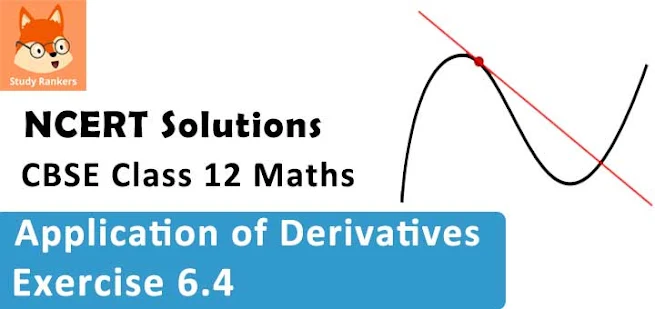### Application of Derivatives Exercise 6.4 Solutions

1. Using differentials, find the approximate value of the following up to 3 places of decimal
(i) √25.3
(ii) √49.5
(iii) √0.6
(iv) (0.009)1/3
(v) (0.999)1/10
(vi) (15)1/4
(vii) (26)1/3
(viii) (255)1/4
(ix) (82)1/4
(x) (401)1/2
(xi) (0.0037)1/2
(xii) (26.57)1/3
(xiii) (81.5)1/4
(xiv) (3.968)3/2
(xv) (32.15)1/5

Solution

(i) √25.3
Consider y = √x . Let x = 25 and Δx = 0.3
Then,
Δy = √(x + Δx) - √x = √(25.3) - √(25) =  √25.3 - 5
⇒ √25.3 = Δy + 5
Now, dy is approximately equal to Δy and is given by,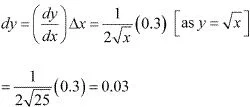Hence, the approximate value of √25.3 is 0.03 + 5 = 5.03.

(ii) √49.5
Consider y = √x . Let x = 49 and Δx = 0.5
Then,
Δy = √(x + Δx) - √x = √(49.5) - √(49) =  √49.5 - 7
⇒ √49.5 = 7 + Δy
Now, dy is approximately equal to Δy and is given by,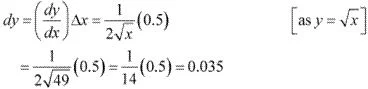Hence, the approximate value of √49.5 is 7 + 0.035 = 7.035

(iii) √0.6
Consider y = √x.
Let x  = 1 and Δx = -0.4
Then,
Δy = √(x + Δx) - √x = √0.6 - 1
⇒ √0.6 = 1 + Δy
Now, dy is approximately equal to Δy and is given by,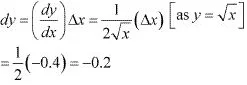Hence, the approximate value of √0.6 is 1 + (-0.2) = 1 - 0.2 = 0.8

(iv) (0.009)1/3
Consider y = x1/3.
Let x = 0.008 and ∆x = 0.001
Then,
Δy = (x + Δx)1/3 - (x)1/3 = (0.009)1/3 - (0.008)1/3 = (0.009)1/3 - 0.2
⇒ (0.009)1/3 = 0.2 + Δy
Now, dy is approximately equal to Δy and is given by,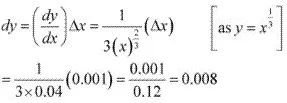Hence, the approximate value of (0.009)1/3 is 0.2 + 0.008 = 0.208

(v) (0.999)1/10
Consider y = (x)1/10.
Let x = 1 and Δx = -0.001.
Then,
∆y = (x + ∆x)1/10 - (x)1/10 = (0.999)1/10 - 1
⇒ (0.999)1/10 = 1 + Δy
Now, dy is approximately equal to ∆y and is given by,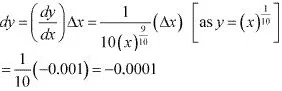Hence, the approximate value of (0.999)1/10 is 1 + (-0.0001) = 0.9999.

(vi) (15)1/4
Consider y = x1/4.
Let x = 16 and Δx = -1.
Then,
∆y = (x + ∆x)1/4 - (x)1/4 = (15)1/4 - (16)1/4 = (15)1/4 - 2
⇒ (15)1/4 = 2 + Δy
Now, dy is approximately equal to Δy and is given by,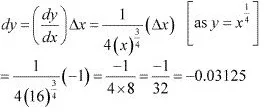Hence, the approximate value of (15)1/4 is 2 + (-0.03125) = 1.96875

(vii) (26)1/3
Consider y = (x)1/3.
Let x = 27 and Δx = -1.
Then,
∆y = (x + ∆x)1/3 - (x)1/3 = (26)1/3 - (27)1/3 = (26)1/3 - 3
⇒ (26)1/3 = 3 + Δy
Now, dy is approximately equal to ∆y and is given by,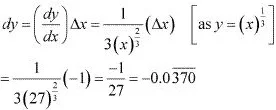Hence, the approximate value of (26)1/3 is 3 + (-0.0370) = 2.9629

(viii) (255)1/4
Consider y = (x)1/4.
Let x = 256 and ∆x = -1
Then,
∆y = (x + ∆x)1/4 - (x)1/4 = (255)1/4 - (256)1/4 = (255)1/4 - 4
⇒ (255)1/4 = 4 + Δy
Now, dy is approximately equal to ∆y and is given by,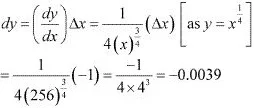Hence, the approximate value of (255)1/4 is 4 + (-0.0039) = 3.9961

(ix) (82)1/4
Consider y = x1/4.
Let x = 81 and Δx = 1.
Then,
∆y = (x + ∆x)1/4 - (x)1/4 = (82)1/4 - (81)1/4 = (82)1/4 - 3
⇒ (82)1/4 =  Δy + 3
Now, dy is approximately equal to ∆y and is given by,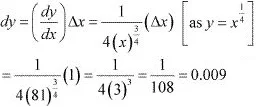Hence, the approximate value of (82)1/4 is 3 + 0.009 = 3.009

(x) (401)1/2
Consider y = x1/2
Let x = 400 and ∆x = 1.
Then,
∆y = √(x + ∆x) - √x = √401 - √400 = √401 - 20
⇒ √401 = 20 + ∆y
Now, dy is approximately equal to ∆y and is given by,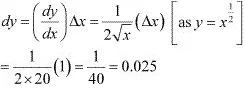Hence, the approximate value of √401 is  20 +0.025 = 20.025

(xi) (0.0037)1/2
Consider y = x1/2
Let x = 0.0036 and ∆x = 0.0001.
Then,
∆y = (x + Δx)1/2 - (x)1/2 = (0.0037)1/2 - (0.0036)1/2 = (0.0037)1/2 - 0.06
⇒ (0.0037)1/2 = 0.06 + Δy
Now, dy is approximately equal to Δy and is given by,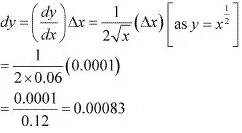Thus, the approximate value of (0.0037)1/2 is 0.06 + 0.00083 = 0.06083.

(xii) (26.57)1/3
Consider y = x1/3.
Let x = 27 and Δx = -0.43
Then,
∆y = (x + Δx)1/3 - (x)1/3 = (26.57)1/3 - (27)1/3 = (26.57)1/3 - 3
⇒ (26.57)1/3 = 3 + Δy
Now, dy is approximately equal to Δy and is given by,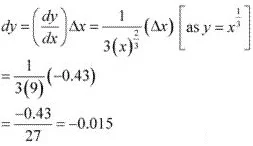Hence, the approximate value of (26.57)1/3 is 3 + (-0.015) = 2.984

(xiii) (81.5)1/4
Consider y = x1/4.
Let x = 81 and Δx = 0.5
Then,
∆y = (x + Δx)1/4 - (x)1/4 = (81.5)1/4 - (81)1/4 = (81.5)1/4 - 3
⇒ (81.5)1/4 = 3 + Δy
Now, dy is approximately equal to Δy  and is given by,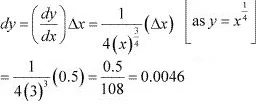Hence, the approximate value of (81.5)1/4 is 3 + 0.0046 = 3.0046

(xiv) (3.968)3/2
Consider y = x3/2,
Let x = 4 and Δx = - 0.032.
Then,
∆y = (x + Δx)3/2 - (x)3/2 = (3.968)3/2  - (4)3/2  = (3.968)3/2  - 8
⇒ (3.968)3/2  = 8 + Δy
Now, dy is approximately equal to ∆y and is given by,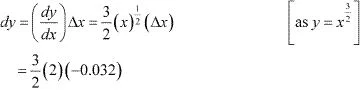= -0.096
Hence, the approximate value of (3.968)3/2 is 8 + (-0.096) = 7.904

(xv) (32.15)1/5
Consider y = x1/5.
Let x = 32 and Δx = 0.15
Then,
∆y = (x + Δx)1/5 - (x)1/5 = (32.15)1/5 - (32)1/5 = (32.15)1/5 - 2
⇒ (32.15)1/5 = 2 + Δy
Now, dy is approximately equal to Δy and is given by,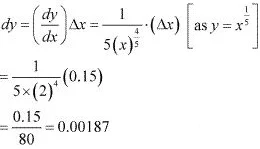Hence, the approximate value of (32.15)1/5 is 2 + 0.00187 = 2.00187

2. Find the approximate value of f (2.01), where f (x) = 4x2 + 5x + 2.
Solution
Let x = 2 and ∆x = 0.01 Then, we have :
f(2.01) = f(x + ∆x) = 4(x + ∆x)2 + 5(x + Δx) + 2
Now, Δy = f(x + Δx) - f(x)
∴ f(x + Δx) = f(x) + Δx
≈f(x) + f '(x).Δx     (as dx = Δx)
⇒ f(2.01) ≈ (4x2 + 5x + 2) + (8x + 5)Δx
= [4(2)2 + 5(2) + 2] + [8(2) + 5](0.01)  [as x = 2, Δx = 0.01]
= (16 + 10 + 2) + (16+5)(0.01)
= 28 + (21)(0.01)
= 28 + 0.21
= 28.21
Hence, the approximate value of f(2.01) is 28.21.

3. Find the approximate value of f (5.001), where f (x) = x3 − 7x2 + 15.
Solution
Let x = 5 and Δx = 0.001. Then, we have :
f(5.001) = f(x + ∆x) = (x + ∆x)3 - 7(x + Δx)2 + 15
Now, Δy = f(x + Δx) - f(x)
∴ f(x + Δx) = f(x) + Δy
≈ f(x) + f '(x).Δx     (as dx = Δx)
⇒ f(5.001) ≈ (x3 - 7x2  + 15) + (3x2 - 14x)Δx
= [(5)3 + 7(5)2  + 15] + [3(5)2  - 14(5)](0.001)  [x = 5, Δx = 0.001]
= (125 - 175 + 15) + (75 - 70) (0.001)
= -35 + (5)(0.001)
= -35 + 0.005
= -34.995
Hence, the approximate value of f(5.001) is -34.995.

4. Find the approximate change in the volume V of a cube of side x metres caused by increasing side by 1%.
Solution
The volume of a cube (V) of side x is given by V = x3 .
∴ (dv/dx) Δx
= (3x2 )Δx
= (3x2 )(0.01x)  [as 1% of x is 0.01x]
= 0.03x3
Hence, the approximate change in the volume of the cube is 0.03x3 m3 .

5. Find the approximate change in the surface area of a cube of side x metres caused by decreasing the side by 1% .
Solution
The surface area of a cube (S) of side x is given by S = 6x2 .
∴ dS = (dS/dx) Δx
= (12x) Δx
= (12x) (-0.01x)  [as 1% of x is 0.01 x]
= -0.12x2
Hence, the approximate change in the surface area of the cube is -0.12x2 m2

6. If the radius of a sphere is measured as 7 m with an error of 0.02m, then find the approximate error in calculating its volume.
Solution
Let r be the radius of the sphere and Δ r be the error in measuring the radius.
Then,
r = 7m and Δr = 0.02 m
Now; the volume V of the sphere is given by,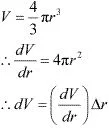= (4πr2 ) ∆r
= 4π(7)2 (0.02)m3 = 3.92π m3
Hence, the approximate error in calculating the volume is 3.92 πm3 .

7. If the radius of a sphere is measured as 7 m with an error of 0.02m, then find the approximate error in calculating its volume.
Solution
Let r be the radius of the sphere and Δr be the error in measuring the radius.
Then,
r = 9 m and Δr = 0.03 m
Now, the surface area of the sphere (S) is given by,
S = 4πr2
∴ dS/dr = 8πr
∴ dS = (dS/dr) Δr
= (8πr)Δr
= 8π(9)(0.03) m2
= 2.16π m2
Hence, the approximate error in calculating the surface area is 2.16π m2 .

8. If f (x) = 3x2 + 15x + 5, then the approximate value of f (3.02) is
A. 47.66
B. 57.66
C. 67.66
D. 77.66
Solution
Let x = 3 and Δx = 0.02. Then, we have :
f(3.02) = f(x + Δx) = 3(x + Δx)2 + 15(x + ∆x) + 5
Now, ∆y = f(x + ∆x) - f(x)
⇒ f(x + ∆x) = f(x) + ∆y
≈ f(x) + f '(x) ∆x      (As dx = ∆x)
⇒ f(3.02) ≈ (3x2 + 15x + 5) + (6x + 15)∆x
= [3(3)2 + 15(3) + 5] + [6(3) + 15](0.02)    [As x = 3, Δx = 0.02]
= (27 + 45 + 5) + (18 + 15)(0.02)
= 77+ 33× 0.02
= 77 + 0.66
= 77.66
Hence, the approximate value of f(3.02) is 77.66